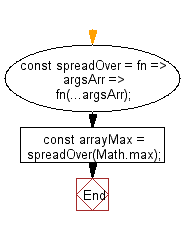# JavaScript: Takes a variadic function and returns a closure that accepts an array of arguments to map to the inputs of the function

## JavaScript fundamental (ES6 Syntax) exercises, practice and solution: Exercise-138 with Solution

Write a JavaScript program that takes a variadic function and returns a closure that accepts an array of arguments to map to the inputs of the function.

Note: Use closures and the spread operator (...) to map the array of arguments to the inputs of the function.

• Use a closure and the spread operator (...) to map the array of arguments to the inputs of the function.

Sample Solution:

JavaScript Code:

``````//#Source https://bit.ly/2neWfJ2
const spreadOver = fn => argsArr => fn(...argsArr);

console.log(arrayMax([1, 2, 3]));
```
```

Sample Output:

```3
```

Pictorial Presentation:Flowchart:Live Demo:

See the Pen javascript-basic-exercise-138-1 by w3resource (@w3resource) on CodePen.

Improve this sample solution and post your code through Disqus

What is the difficulty level of this exercise?

Test your Programming skills with w3resource's quiz.

﻿

## JavaScript: Tips of the Day

Chunks an array into n smaller arrays

Example:

```const tips_chunkIntoN = (arr, n) => {
const size = Math.ceil(arr.length / n);
return Array.from({ length: n }, (v, i) =>
arr.slice(i * size, i * size + size)
);
}
console.log(tips_chunkIntoN([1, 2, 3, 4, 5, 6, 7,8], 4));
```

Output:

```[[1,2],[3,4],[5,6],[7,8]]
```